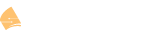# Python Numeric Types

This topic is to discuss the following lesson:

Hi Rene,

In given eg,
my_integer = 1.9
type(my_integer)

my_int = int(my_integer)
type(my_int)

i could not understand the output of last two commands , as per me it will be like this:

``````type(my_integer) =  type(1.9)
my_int = int(1.9)
type(my_int) = type (int(1.9))
``````

so as per you it shows that class is integer(what integer) and How and how it get rid of decimal values?

The code in this particular example is as follows:

`my_integer = 1.9`

The above code creates a variable called `my_integer` and assigns it a value of 1.9. This will automatically make this variable a float type because it is not an integer, but a real number.

`type(my_integer)`

This code causes output that indicates the type of variable that `my_integer` is. You can see this returns the following text: <class ‘float’> so it is verified that the `my_integer` variable is of float type.

`my_int = int(my_integer)`

The above code takes the `my_integer` variable, which is of type float, and converts it to an integer, and stores it in the `my_int` variable. The result is that the `my_int` variable now contains an integer of value 1. Remember that whenever a float type is converted to an integer type, the result is a “floored” value, meaning any decimal digits are simply removed.

If you add the following code, you can print out the value of the `my_int` variable:

print(my_int)

From the below screenshot you can see that this value is indeed 1.

`type(my_int)`

Finally, the above code returns the type of variable that `my_int` is which is an integer.

I hope this has been helpful!

Laz